# O ELECTROCHEMISTRY Recognizing consistency among equilibrium constant, free... A student made measurements on some electrochemical cells...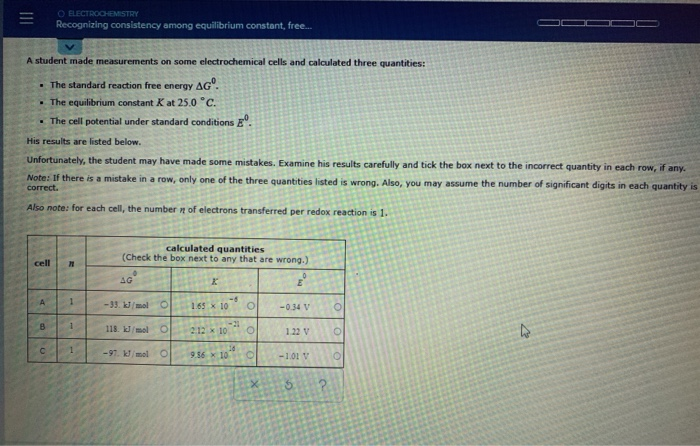O ELECTROCHEMISTRY Recognizing consistency among equilibrium constant, free... A student made measurements on some electrochemical cells and calculated three quantities: . The standard reaction free energy AGO. . The equilibrium constant Kat 25.0 °C. • The cell potential under standard conditions E. His results are listed below. Unfortunately, the student may have made some mistakes. Examine his results carefully and tick the box next to the incorrect quantity in each row, if any. Note: If there is a mistake in a row, only one of the three quantities listed is wrong. Also, you may assume the number of significant digits in each quantity is correct. Also note: for each cell, the number of electrons transferred per redox reaction is 1. cell calculated quantities (Check the box next to any that are wrong.) AG A 1 - 33 kJ/mol 1.65 x 10 O -0.34 118. kJ/mol O 1.22 V -21 2.12 x 10 10 9.56 x 10 с -97 kJ/mol -1.01 \$
Show transcribed image text

This Homework Help Question: "O ELECTROCHEMISTRY Recognizing consistency among equilibrium constant, free... A student made measurements on some electrochemical cells..." No answers yet.

We need 10 more requests to produce the answer to this homework help question. Share with your friends to get the answer faster!

0 /10 have requested the answer to this homework help question.

Once 10 people have made a request, the answer to this question will be available in 1-2 days.
All students who have requested the answer will be notified once they are available.
##### Add Answer of: O ELECTROCHEMISTRY Recognizing consistency among equilibrium constant, free... A student made measurements on some electrochemical cells...
Similar Homework Help Questions
• ### III O ELECTROCHEMISTRY Recognizing consistency among equilibrium constant, free... A student made measurements on some electrochemical...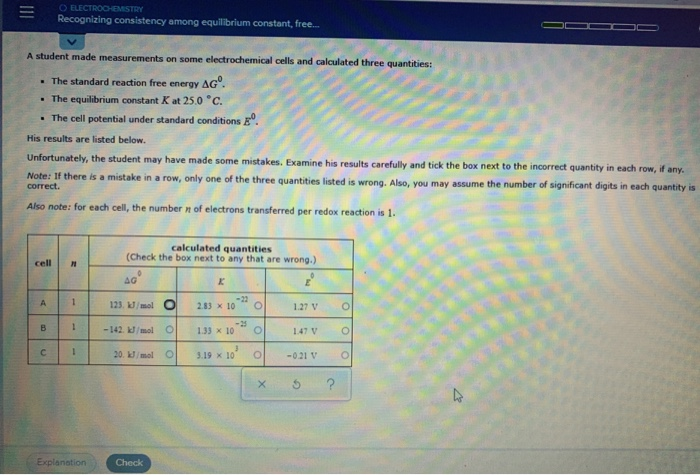III O ELECTROCHEMISTRY Recognizing consistency among equilibrium constant, free... A student made measurements on some electrochemical cells and calculated three quantities: . The standard reaction free energy Agº. . The equilibrium constant Kat 25.0*c. • The cell potential under standard conditions E. His results are listed below. Unfortunately, the student may have made some mistakes. Examine his results carefully and tick the box next to the incorrect quantity in each row, if any. Note: If there is a mistake in...

• ### ADVANCED MATERIAL Recognizing consistency among equilibrium constant, free energ... A student made measurements on some electrochemical...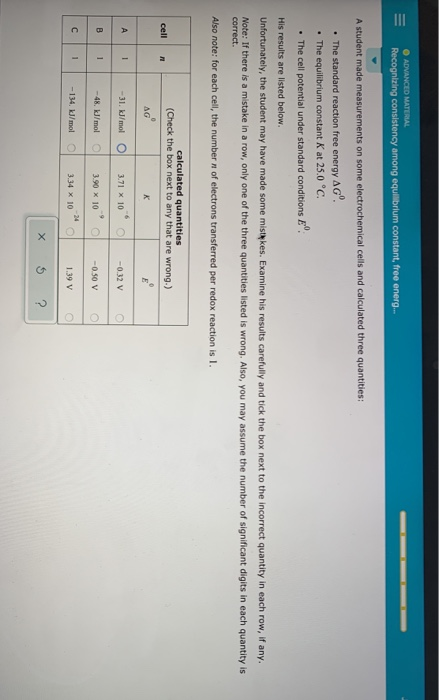ADVANCED MATERIAL Recognizing consistency among equilibrium constant, free energ... A student made measurements on some electrochemical cells and calculated three quantities: • The standard reaction free energy AG". • The equilibrium constant K at 25.0°C. . The cell potential under standard conditions E. His results are listed below. Unfortunately, the student may have made some mistes. Examine his results carefully and tick the box next to the incorrect quantity in each row, if any. Note: If there is a mistake...

• ### O ADVANCED MATERIAL Recognizing consistency among equilibrium constant, free energ... A student made measurements on some...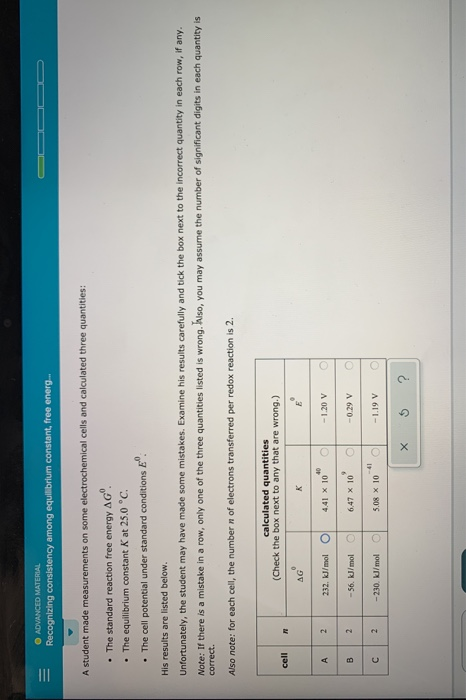O ADVANCED MATERIAL Recognizing consistency among equilibrium constant, free energ... A student made measurements on some electrochemical cells and calculated three quantities: • The standard reaction free energy AG". • The equilibrium constant K at 25.0 °C. • The cell potential under standard conditions E". His results are listed below. Unfortunately, the student may have made some mistakes. Examine his results carefully and tick the box next to the incorrect quantity in each row, if any. Note: If there is...

• ### ADVANCED MATERIAL Recognizing consistency among equilibrium constant, free energ... * A student made measurements o...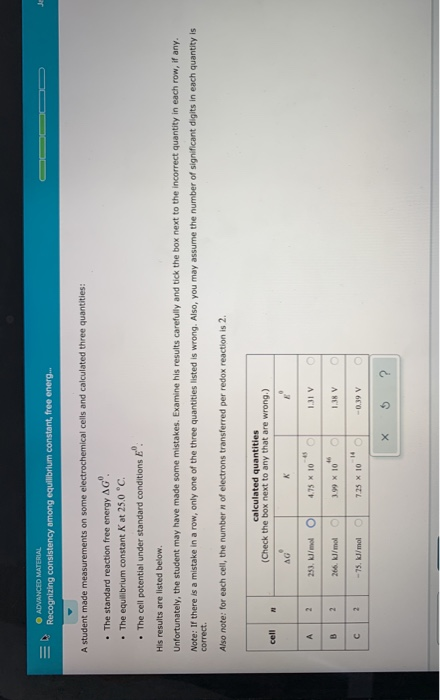ADVANCED MATERIAL Recognizing consistency among equilibrium constant, free energ... * A student made measurements on some electrochemical cells and calculated three quantities: . The standard reaction free energy AG'. . The equilibrium constant K at 25.0 °C. • The cell potential under standard conditions E". His results are listed below. Unfortunately, the student may have made some mistakes. Examine his results carefully and tick the box next to the incorrect quantity in each row, if any. Note: If there is...

• ### Help! Not sure how to answer this whole question O ELECTROCHEMISTRY Recognizing consistency among equilibrium constant,...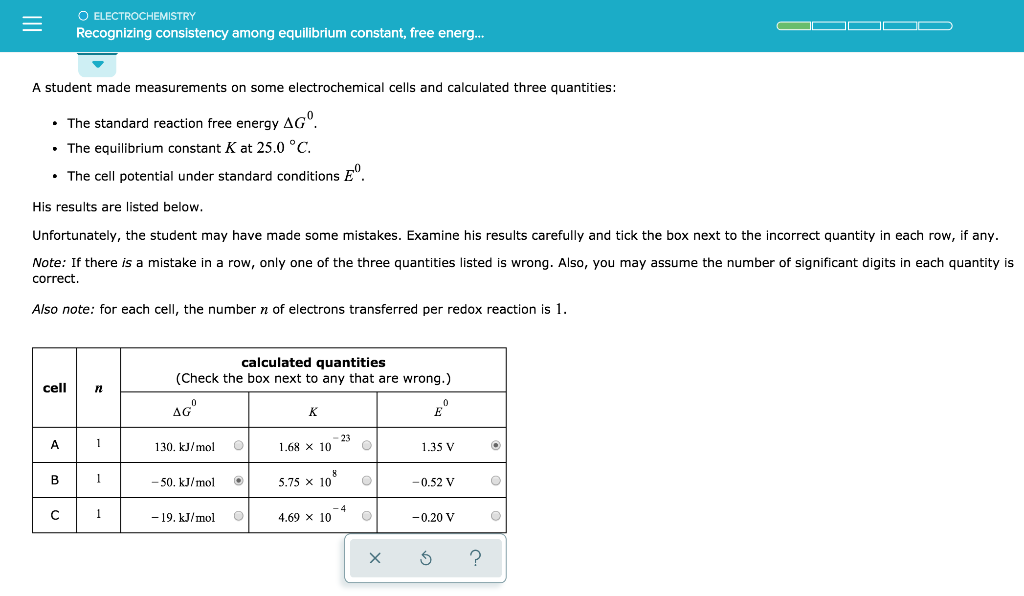Help! Not sure how to answer this whole question O ELECTROCHEMISTRY Recognizing consistency among equilibrium constant, free energ... A student made measurements on some electrochemical cells and calculated three quantities: • The standard reaction free energy AG'. • The equilibrium constant K at 25.0 °C. • The cell potential under standard conditions E. His results are listed below. Unfortunately, the student may have made some mistakes. Examine his results carefully and tick the box next to the incorrect quantity in...

• ### A student made measurements on some electrochemical cells and calculated three quantities: • The standard reaction...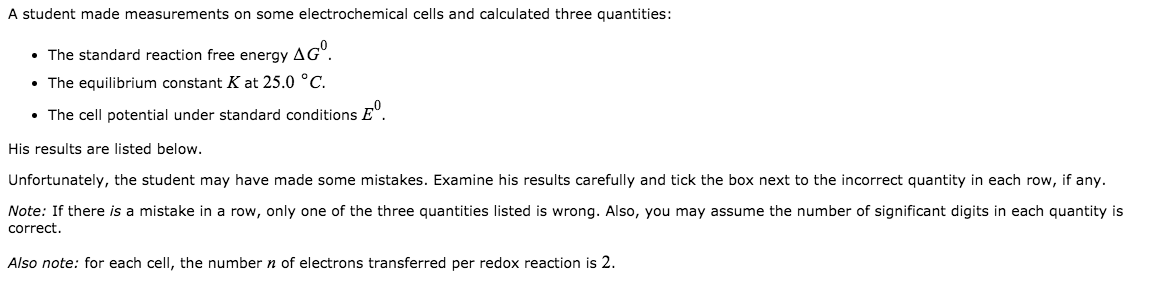A student made measurements on some electrochemical cells and calculated three quantities: • The standard reaction free energy AG'. • The equilibrium constant K at 25.0 °C. • The cell potential under standard conditions E. His results are listed below. Unfortunately, the student may have made some mistakes. Examine his results carefully and tick the box next to the incorrect quantity in each row, if any. Note: If there is a mistake in a row, only one of the three...

• ### Part A: Part B: Recognizing consistency among equilibrium constant, free... A student made measurements on some...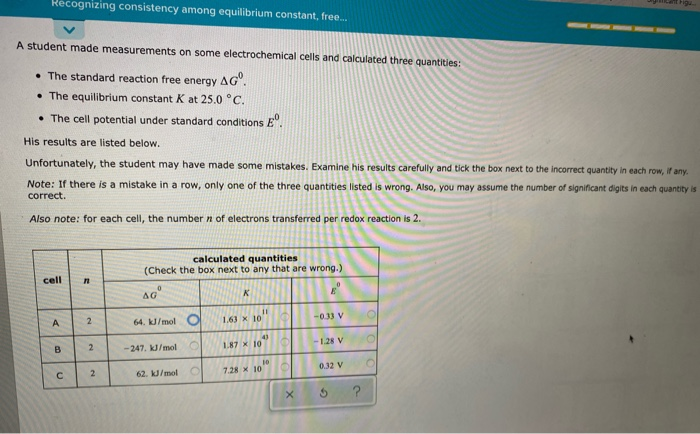Part A: Part B: Recognizing consistency among equilibrium constant, free... A student made measurements on some electrochemical cells and calculated three quantities: • The standard reaction free energy AG'. . The equilibrium constant K at 25.0 °C. • The cell potential under standard conditions eº. His results are listed below. Unfortunately, the student may have made some mistakes. Examine his results carefully and tick the box next to the incorrect quantity in each row, if any. Note: If there is...

• ### A student made measurements on some electrochemical cells and calculated three quantities 0 The standard reaction free energy AG . The equilibrium constant K at 25.0 °C 0 The cell potential under sta...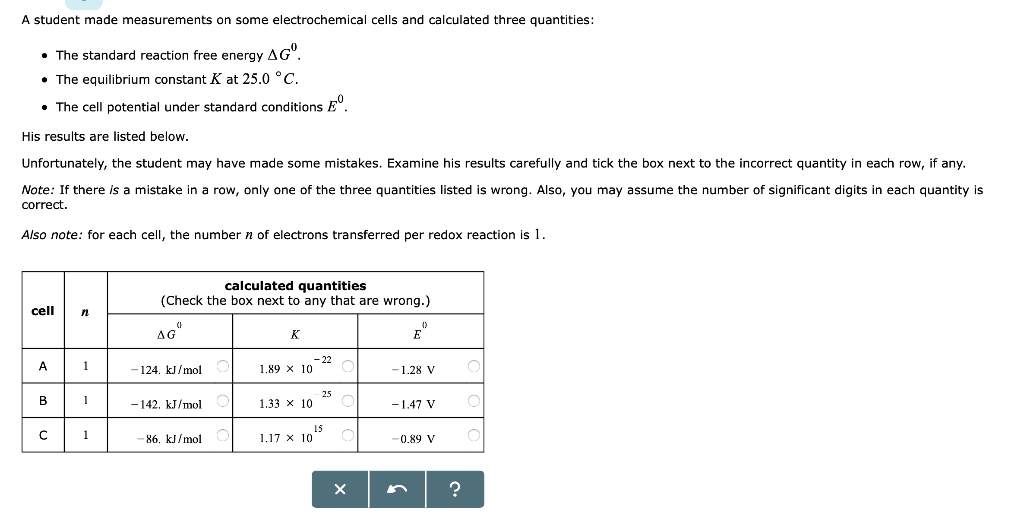A student made measurements on some electrochemical cells and calculated three quantities 0 The standard reaction free energy AG . The equilibrium constant K at 25.0 °C 0 The cell potential under standard conditions E. His results are listed below Unfortunately, the student may have made some mistakes. Examine his results carefully and tick the box next to the incorrect quantity in each row, if any Note: If there is a mistake in a row, only one of the three...

• ### - Recognizing consistency among equilibrium constant, free... . The standard reaction free energy AGO. . The...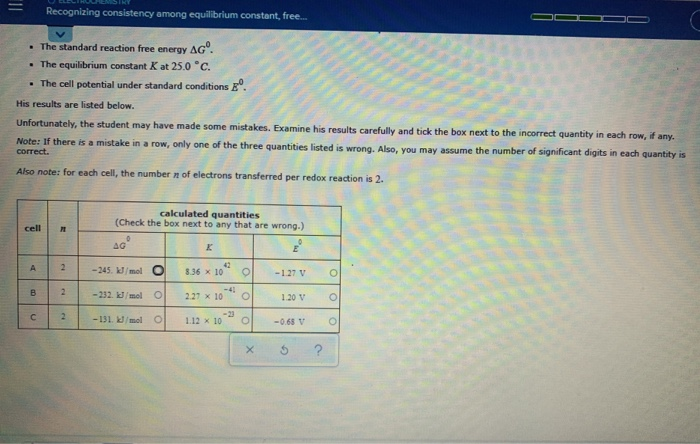- Recognizing consistency among equilibrium constant, free... . The standard reaction free energy AGO. . The equilibrium constant K at 25.0 °C. • The cell potential under standard conditions E. His results are listed below. Unfortunately, the student may have made some mistakes. Examine his results carefully and tick the box next to the incorrect quantity in each row, if any. Note: If there is a mistake in a row, only one of the three quantities listed is wrong. Also,...

• ### A student made measurements on some electrochemical cells and calculated three quantities: • The standard reaction...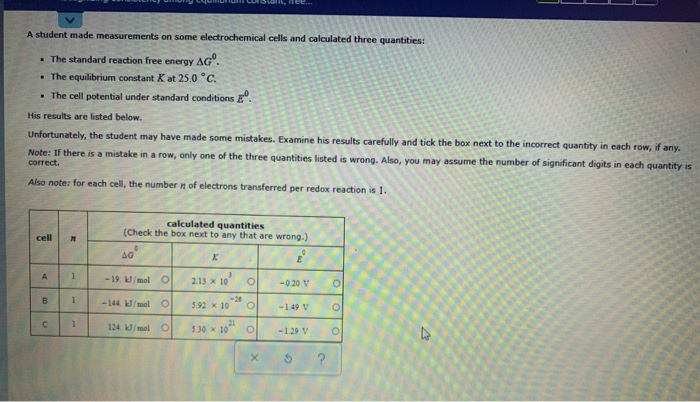A student made measurements on some electrochemical cells and calculated three quantities: • The standard reaction free energy AGO. • The equilibrium constant K at 25.0°c. • The cell potential under standard conditions E. His results are listed below. Unfortunately, the student may have made some mistakes. Examine his results carefully and tick the box next to the incorrect quantity in each row, if any. Note: If there is a mistake in a row, only one of the three quantities...

Free Homework App